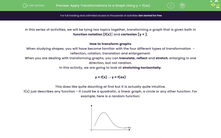# Apply Transformations to a Graph Using y = f(ax)

In this worksheet, students will practise applying the transformation y = f(ax) to a function defined as y = f(x).Key stage:  KS 4

Year:  GCSE

GCSE Subjects:   Maths

GCSE Boards:   AQA, Eduqas, OCR, Pearson Edexcel,

Curriculum topic:   Algebra, Graphs of Equations and Functions

Curriculum subtopic:   Graphs Transformation of Curves and Their Equations

Difficulty level:#### Worksheet Overview

In this series of activities, we will be tying two topics together, transforming a graph that is given both in function notation [f(x)] and cartesian [y = ].

How to transform graphs

When studying shapes, you will have become familiar with the four different types of transformation  - reflection, rotation, translation and enlargement.

When you are dealing with transforming graphs, you can translate, reflect and stretch, enlarging in one direction, but not rotation.

In this activity, we are going to look at stretching horizontally.

y = f(x) → y = f(ax)

This does like quite daunting at first but it is actually quite intuitive.

f(x) just describes any function - it could be a quadratic, a linear graph, a circle or any other function. For example, here is a random function:If we apply the transformation y = f(ax), we are multiplying each value of x by a (which could be any value) before we evaluate the function.

This has the effect of giving a specific value of y before it would happen in the original function.

While this sounds incredibly confusing, what it means is that the graph stretches horizontally.

You need to remember that a transformation of y = f(ax) stretches the graph horizontally with a scale factor of 1/a.

To stretch the function out horizontally, the value of a would have to be fractional.The function y = f(ax) is a stretch in a horizontal direction with a scale factor of 1/a.

How to apply this to a graph

Example:

You are given the function y = x2 - 4

Sketch the graph of y = (2x2) – 4

When we are looking at this transformation, you need to notice that the multiplier by 2 is inside the bracket with the x - this makes it a horizontal stretch.

In the transformation y = f(2x), a = 2 so every value of x will halve (a scale factor of ½) but the values of y will stay the same.Sometimes when you translate a function, you will be expected to label the significant points that have been translated.

This only needs to be done if it is specifically asked for.

Let's move on to some questions.

### What is EdPlace?

We're your National Curriculum aligned online education content provider helping each child succeed in English, maths and science from year 1 to GCSE. With an EdPlace account you’ll be able to track and measure progress, helping each child achieve their best. We build confidence and attainment by personalising each child’s learning at a level that suits them.

Get started